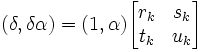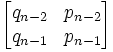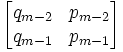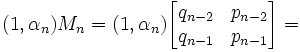## Wednesday, January 04, 2006

### Continued Fractions and Matrices: More Lemmas

Today's blog continues exploring properties of continued fractions and matrices which are used in the solution for Pell's Equation. This blog assumes that you have already read the previous blog.

The details of today's blog is based on Harold M. Stark's An Introduction to Number Theory.

Lemma 1: if δ(1,√d) = (1,√d)Mn-1Mn+kj, then there exists rk, sk, tk, uk such that:and

(dtk - sk) + (rk - uk)√d = 0.

(1) By assumption, we let:
δ(1,√d) = (1,√d)Mn-1Mn+kj [See here for an example where this assumption is true]

(2) Further, since we know that Mn-1 is a 2 x 2 matrix (see here), we know that the product with Mn+kj is a 2x2 matrix (see here) and we can assume that there exists rk, sk, tk, uk such that:(3) Now, we know that:
δ(1,α) = (δ,δα) [See here for review of matrix math]

(4) From a previous result, we know that:
δ(1,α) = (1,α)Mn-1Mn+j [See here]

(5) Combining #4 and #5 with #2 gives us:(6) From #6 (see here for review of matrix math), we can conclude that:

(δ, δα) = (rk+tkα, sk+ukα)

(7) This means that:

δ = rk + tkα

δα = sk + ukα

(8) Combining these two equations gives:

(rk + tkα)α = sk + ukα

(9) Which means that:

rkα + tkα2 = sk + ukα

(10) And subtracting the left-side value from both sides give us:

tkα2 + rkα - ukα - sk = 0

which is:

tkα2 + α(rk - uk) - sk = 0

(11) Since we know that α = √d (see here for review of α)

tkα2 + α(rk - uk) - sk = tkd +√d (rk - uk) - sk

(12) So, this gives us finally:
(dtk - sk) + (rk - uk)√d= 0.

QED

Lemma 2: Mn = Mm → n = m.

(1) Assume that Mn = Mm but n ≠ m

(2) Mn =(3) Mm =(4) Since Mn = Mm, we have:

qn-2 = qm-2
qn-1 = qm-1

(5) Now q1 is less than q2 and so on (see here for proof). So, m-2, n-2, n-1, m-1 must all be less than 1. (Otherwise, they cannot be equal)

(6) q0 and q-2 = 1 while q-1 = 0.

(7) So, m-2, n-2, n-1, m-1 cannot equal -1. [Since by #6 no other value = 0]

(8) On the other, it is possible for q-2 = q0 = q1 since all could = 1. (See here and here)

(9) So n-2, m-2, n-1, m-1 must equal -2,0, or 1.

(10) So n must = 2 (so that n-1 = 1, n-2 = 0). [Note: n=0 doesn't work since n-1 = -1; n=1 doesn't work since n-2=-1; and n=3 doesn't work since n-1 = 2]

(11) But then m must also = 2 (so that m-1=1, m-2=0) but this goes against our assumption.

(12) So we have a contradiction. There is no way that Mn = Mm without n = m.

QED

### Continued Fractions and Matrices Continued

Today's blog continues a previous blog on how 2x2 matrices can be used to represent continued fractions. The lemmas presented in today's blog are used in the solution to Pell's Equation. For those interested in the background to Pell's Equation, start here. For those who would like to proceed to its solution using continued fractions and matrices, go here. The solution to Pell's Equation is used as part of the proof for Fermat's Last Theorem, n=5.

The content in today's blog is based on Harold M. Stark's An Introduction to Number Theory.

For Lemma 1, αn, qn are defined here. For Lemma 2, Mn is defined here.

Lemma 1: αnqn-1 + qn-2 ≠ 0

(1) Assume that αnqn-1 + qn-2 = 0.

(2) Then, qn-1 = 0 and qn-2 = 0. [See here for proof]

(3) But qn ≥ 1 for n ≥ 1. [See here]

(4) Futher, there are no successive 0's in a row since (see here for details on how these values are derived):
q-2 = 1
q-1=0
q0=1

(5) Therefore #2 is impossible and we reject our assumption.

QED

Lemma 2: if γn = αnqn-1 + qn-2, then γn(1,α) = (1,αn)Mn

(1) From the definition of Mn (see here), we know that:= (qn-2nqn-1, pn-2npn-1) [See here for review on matrix products]

(2) By applying the equation α = (pn-2 + αnpn-1)/(qn-2 + αnqn-1) [See here], we get:

(qn-2nqn-1 , pn-2npn-1) = (qn-2 + αnqn-1)(1,α)

(3) Adding back γn, this gives us:
(qn-2 + αnqn-1)(1,α) = γn(1,α)

QED

Lemma 3: if δ = γn+jn, then δ(1,√d) = (1,√d)Mn-1Mn+kj

(1) From Lemma 2, we know that;

γn(1 , α) = (1, αn)Mn

and

γn+j(1, α) = (1, αn+j)Mn+j

(2) By the definition of matrix inverses (see here), we know that:
(1,αn)MnMn-1 = (1,αn)

(3) From #1, then we have:
[(1,αn)Mn)] = γn(1,α)

(4) So, from #2, we have:
(1,αn) = γn(1,α)Mn-1

(5) Likewise since αn = αn+j (see here), we get:
(1,αn+j)Mn+j = (1,αn)Mn+j

(6) Applying #4, this gives us:
(1,αn+j)Mn+j = γn(1,α)Mn-1Mn+j

(7) Combining #1 with #6, gives us:
γn+j(1,α) = γn(1,α)Mn-1Mn+j

(8) Now, δ = γn+jn [We can do this since γn ≠ 0 (see Lemma 1 above) ]

(9) δ(1,α) = [(γn+j)(1 , α)]/γn

(10) Applying #7 gives us;

δ(1,α) = [γn(1,α)Mn-1Mn+j]/γn =
= (1,α)Mn-1Mn+j

QED